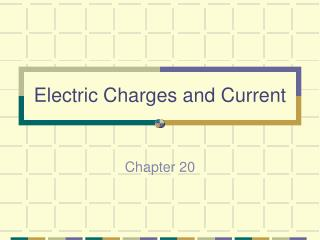DownloadDownload PresentationElectric Charges and Current

# Electric Charges and Current

Download Presentation## Electric Charges and Current

- - - - - - - - - - - - - - - - - - - - - - - - - - - E N D - - - - - - - - - - - - - - - - - - - - - - - - - - -
##### Presentation Transcript

1. Electric Charges and Current Chapter 20

2. Electric Charge • proton - positive charge • electron - negative charge • Charges that are the same repel each other. • Charges that are different attract each other. Chapter 20

3. Electric field - the region around charged particles through which a force is exerted. • electric field lines pg. 646 Chapter 20

4. Static Electricity • A buildup of charges on an object. • In static electricity, charges build up, but they do not flow • transferring charge - friction, conduction & induction (overhead) • static cling - fabrics rub together • when a negatively charged object and a positively charged object are brought together, electrons move until both objects have the same charge. Chapter 20

5. Lightning - discharge of static electricity • electroscope - instrument used to detect electric charge Chapter 20

6. Circuit Measurements • electric potential - potential energy per unit of electric charge • voltage - difference in electrical potential between two points • electricity flows from higher potential to lower potential • voltage causes current to flow through an electric circuit • voltage sources - battery and generators Chapter 20

7. just as an increase in the difference in height causes a greater flow of water, an increase in voltage causes a greater flow of electric current pg.656 • the greater the resistance, the less current there is for a given voltage. • Long/short • thick/thin wires • electricity will take the path of least resistance • birds on a wire Chapter 20

8. voltmeter - measures voltage • ammeter - measures current • Voltage = current x resistance V=IR • Sample problems pg.659 Chapter 20

9. Series and Parallel Circuits • series circuit - there is only one path for the current to take • one bulb out - all out, • resistance increases with more bulbs • parallel circuit - there are several paths for current to take • one bulb out - others on, • resistance decreases • household circuits in parallel Chapter 20

10. Electrical Safety • short circuit - a connection that allows current to take an unintended path • damaged insulation, water on floor, barefoot • three prong plugs - electricity flows to ground instead of person if short circuit occurs Chapter 20

11. when lightning strikes the lightning rod, charges flow through the rod, into the wire and then into the Earth Chapter 20

12. electrical current can become to high if a circuit is overloaded. In order to prevent circuits from overheating, devices called fuses and circuit breakers are added to circuits. Open circuits when overload • the severity of electric shock depends on the current • electric safety pg.670 Chapter 20